The WinTess software checks if a structure properly supports the loads applied using the “Ratio” concept.

The ratio is a numerical value indicating how close we are or exceed load carrying capacity considering the safety factor of any part of the structure. The value “1” is the maximum limit. If we exceed this value, we understand that part of the structure is undersized. If the value is very small, for example less than 0.4, we can say that this component of the structure is oversized.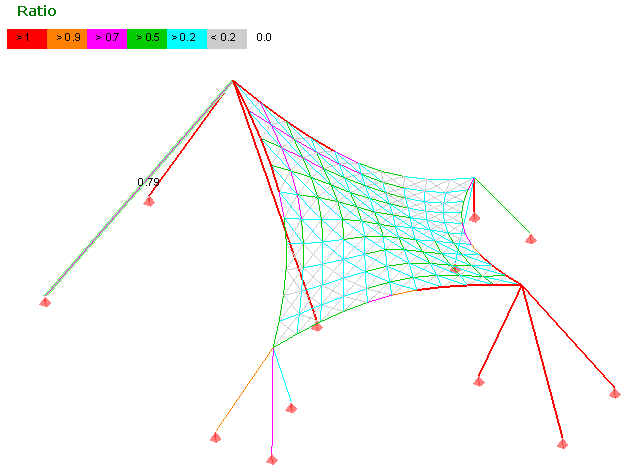The ratio r is obtained using the following formula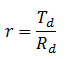where,

Td = Stress on the component

Rd = Resistance of the component

The letter ” d “is often used to indicate that these are design values. However, we must bear in mind that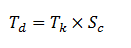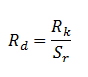where,

Tk = Stress on the component due to loads
Sc = Safety factor on load side
Rk = Resistance of the component
Sr  = Safety factor on material side

So, we obtain equation given below: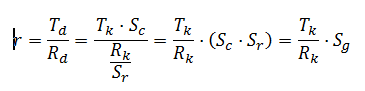If Sg is the global safety factor, then Sg = Sc · Sr

This is equivalent to using safety coefficients such that

Sc = 1                         Sr = Sg

WinTess can calculate ratios using two methods:

• Using the default safety factor values of WinTess. This means Sc = 1 and a global safety factor on material is applied to cover both safety factors on loads and materials.
• Entering Sc and Sr separately.

In addition, WinTess allow you to enter different values of safety factors for each type of action and each type of structural element.

Combinations

What happens when we use different safety factors for different type of actions?

Suppose we have an action 1 with a safety factor Sc1 and another action 2 with a safety factor Sc2. The ratio for this combination of hypotheses will be: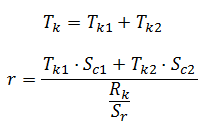In linear calculation where results (deflections, stresses, etc) are proportional to the actions, what we usually do is to calculate the stresses for each type of action (1, 2) separately and obtain Tk1 and Tk2.  Then the ratio r can quickly be obtained through applying the safety factors of Sc1 and Sc2. The stress produced as a result of an action on an element is proportional to action A: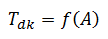Stress depends on action

In this case the same safety factor is applied to the action or stress. As it is proportional in linear calculation, there is a correlation >between actions and effects thereof: doubling the action creates double deformation, double stress, etc.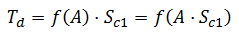In the non-linear calculation, which is the usual situation WinTess this is not as easy as proportional. There is no correlation between the actions and the effects thereof: doubling the action does NOT create double deformation, double stress, etc.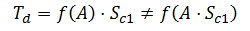Example

Suppose that a structure is subjected to three actions: snow, wind and self weight. Depending on the combination, a different safety factor for each action (load) will be used.

```Combination      Snow          Wind          Self Weight
1            0            0                1.5
2            1.5          0                1.35
3            0            1.5              1.35
4            1.35         1.5              1.35
5            1.5          1.35             1.35```

In the case of linear calculation (typical building structures with concrete, steel, etc.), we calculate the stresses Tk (i) causing each hypothesis separately and then find the stresses of each combination:

Td(1)  =  1.5 · Tk(SelfWeight)  =  Tk(SelfWeight · 1.5)

Td(2)  =  1.5 · Tk(Snow)  +   1.35 · Tk(SelfWeight)  =  Tk(Snow · 1.5)  +  Tk(SelfWeight · 1.35)

etc.

However, if it is non-linear calculation, these formulas are not correct, since

1.5 · Tk(SelfWeight)  ≠  Tk(SelfWeight · 1.5)

1.5 · Tk(Snow)  +  1.35 · Tk(SelfWeight)  ≠  Tk(Snow · 1.5)  +  Tk(SelfWeight · 1.35)

It has no use to calculate stresses of each action separately, as these stresses can not be multiplied by a factor to get the stresses of the combination. Only we can use:

Td(1) = Tk(SelfWeight · 1.5)

Td(2) = Tk(Snow · 1.5) + Tk(SelfWeight · 1.35)

etc.

That is, we repeat the calculation for each combination of actions by multiplying each action with its safety factor. In WinTess, that means creating a new file and apply a combination with factored loads: higher snow load, higher wind speed, etc. Still, the result of the stresses obtained can not be real as it is calculated with a deflection which is not real but factored.

##### Conclusion

However, this is not the ultimate solution. In a non-linear calculation, if we factorize actions, we obtain results that correspond to these actions. But these actions are not real and can lead to erroneous results. We have already said thatThus, to analyze a combination of actions with different safety factors for each of them, we choose one of two solutions given below. However, keep in mind that none of them is fully correct:

Solution 1:

We consider that it is linear calculation . We get the stress Tk for each non factored action. Then, we multiply the safety factor of each action to its corresponding stress, for a total stress Td  which gives us the value of the ratio r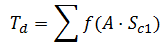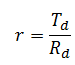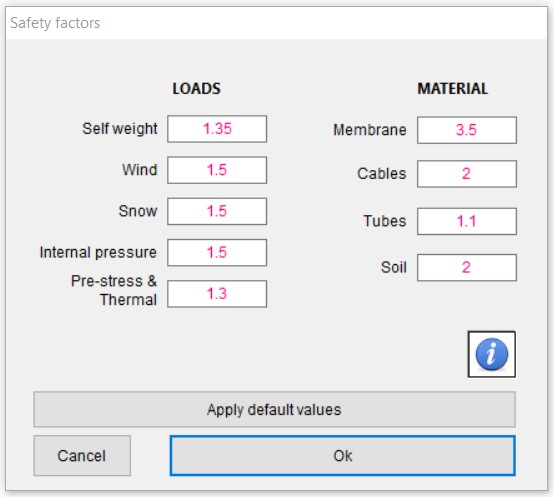Solution 2:

We consider that it is non-linear calculation. We find the stress Tk (i) for each non factored action and add them together to get a total stress Tk. Then we find a global safety factor depending on the safety factor of each action (a calculation judgment), which leads to a ratio value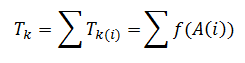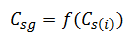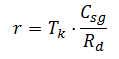We can concentrate on the safety factor Csg for the material: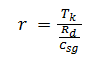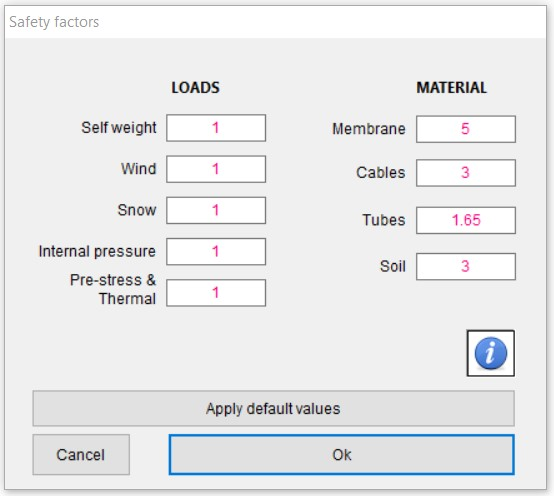Actually this second solution is what WinTess uses when applying safety factors = 1 to the actions and higher safety factors to materials.